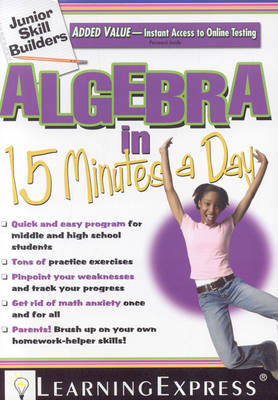•Algebra in 15 Minutes a Day (Paperback)

(other)
£9.99
Paperback 256 Pages / Published: 01/03/2011
• We can order this

Usually dispatched within 2 weeks

You don't have to be a genius to become an algebra ace-you can do it in just 15 minutes a day Packed with short and snappy lessons, Junior Skill Builders: Algebra in 15 Minutes a Day makes learning algebra easy. It's true: making sense of algebra doesn't have to take a long time ...and it doesn't have to be difficult! In just one month, students can gain expertise and ease in all the algebra concepts that often stump students. How? Each lesson gives one small part of the bigger algebra problem, so that every day students build upon what was learned the day before. Fun factoids, catchy memory hooks, and valuable shortcuts make sure that each algebra concept becomes ingrained. With Junior Skill Builders: Algebra in 15 Minutes a Day, before you know it, a struggling student becomes an algebra pro-one step at a time. In just 15 minutes a day, students master both pre-algebra and algebra, including: Fractions, multiplication, division, and other basic math Translating words into variable expressions Linear equations Real numbers Numerical coefficients Inequalities and absolute values Systems of linear equations Powers, exponents, and polynomials Quadratic equations and factoring Rational numbers and proportions And much more! In just 15 minutes a day, students master both pre-algebra and algebra, including: Fractions, multiplication, division, and other basic math Translating words into variable expressions Linear equations Real numbers Numerical coefficients Inequalities and absolute values Systems of linear equations Powers, exponents, and polynomials Quadratic equations and factoring Rational numbers and proportions And much more! In addition to all the essential practice that kids need to ace classroom tests, pop quizzes, class participation, and standardized exams, Junior Skill Builders: Algebra in 15 Minutes a Day provides parents with an easy and accessible way to help their children exce

Publisher: Learning Express Llc
ISBN: 9781576856734
Number of pages: 256
Weight: 467 g
Dimensions: 255 x 183 x 18 mm